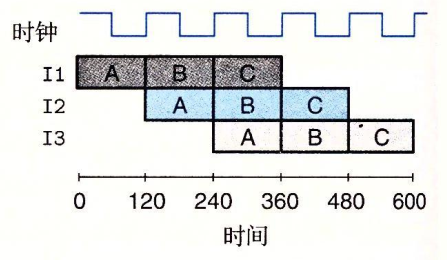# 为什么计算机处理排序数组比未排序数组快?

import java.util.Arrays;
import java.util.Random;

public class Main
{
public static void main(String[] args)
{
// Generate data
int arraySize = 32768;
int data[] = new int[arraySize];

Random rnd = new Random(0);
for (int c = 0; c < arraySize; ++c)
data[c] = rnd.nextInt() % 256;

// !!! With this, the next loop runs faster
Arrays.sort(data);

// Test
long start = System.nanoTime();
long sum = 0;

for (int i = 0; i < 100000; ++i)
{
// Primary loop
for (int c = 0; c < arraySize; ++c)
{
if (data[c] >= 128)
sum += data[c];
}
}

System.out.println((System.nanoTime() - start) / 1000000000.0);
System.out.println("sum = " + sum);
}
}